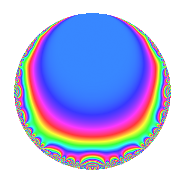# Properties

 Label 189.2.g.aLevel 189 Weight 2 Character orbit 189.g Analytic conductor 1.509 Analytic rank 0 Dimension 2 CM No Inner twists 2

# Learn more about

## Newspace parameters

 Level: $$N$$ = $$189 = 3^{3} \cdot 7$$ Weight: $$k$$ = $$2$$ Character orbit: $$[\chi]$$ = 189.g (of order $$3$$ and degree $$2$$)

## Newform invariants

 Self dual: No Analytic conductor: $$1.5091725982$$ Analytic rank: $$0$$ Dimension: $$2$$ Coefficient field: $$\Q(\sqrt{-3})$$ Coefficient ring: $$\Z[a_1, a_2]$$ Coefficient ring index: $$1$$ Sato-Tate group: $\mathrm{SU}(2)[C_{3}]$

## $q$-expansion

Coefficients of the $$q$$-expansion are expressed in terms of a primitive root of unity $$\zeta_{6}$$. We also show the integral $$q$$-expansion of the trace form.

 $$f(q)$$ $$=$$ $$q$$ $$+ ( 1 - \zeta_{6} ) q^{2}$$ $$+ \zeta_{6} q^{4}$$ $$+ q^{5}$$ $$+ ( -1 + 3 \zeta_{6} ) q^{7}$$ $$+ 3 q^{8}$$ $$+O(q^{10})$$ $$q$$ $$+ ( 1 - \zeta_{6} ) q^{2}$$ $$+ \zeta_{6} q^{4}$$ $$+ q^{5}$$ $$+ ( -1 + 3 \zeta_{6} ) q^{7}$$ $$+ 3 q^{8}$$ $$+ ( 1 - \zeta_{6} ) q^{10}$$ $$-5 q^{11}$$ $$+ ( 5 - 5 \zeta_{6} ) q^{13}$$ $$+ ( 2 + \zeta_{6} ) q^{14}$$ $$+ ( 1 - \zeta_{6} ) q^{16}$$ $$+ ( 3 - 3 \zeta_{6} ) q^{17}$$ $$-\zeta_{6} q^{19}$$ $$+ \zeta_{6} q^{20}$$ $$+ ( -5 + 5 \zeta_{6} ) q^{22}$$ $$-3 q^{23}$$ $$-4 q^{25}$$ $$-5 \zeta_{6} q^{26}$$ $$+ ( -3 + 2 \zeta_{6} ) q^{28}$$ $$-\zeta_{6} q^{29}$$ $$+ 5 \zeta_{6} q^{32}$$ $$-3 \zeta_{6} q^{34}$$ $$+ ( -1 + 3 \zeta_{6} ) q^{35}$$ $$-3 \zeta_{6} q^{37}$$ $$- q^{38}$$ $$+ 3 q^{40}$$ $$+ ( -5 + 5 \zeta_{6} ) q^{41}$$ $$+ \zeta_{6} q^{43}$$ $$-5 \zeta_{6} q^{44}$$ $$+ ( -3 + 3 \zeta_{6} ) q^{46}$$ $$+ ( -8 + 3 \zeta_{6} ) q^{49}$$ $$+ ( -4 + 4 \zeta_{6} ) q^{50}$$ $$+ 5 q^{52}$$ $$+ ( -9 + 9 \zeta_{6} ) q^{53}$$ $$-5 q^{55}$$ $$+ ( -3 + 9 \zeta_{6} ) q^{56}$$ $$- q^{58}$$ $$+ ( 14 - 14 \zeta_{6} ) q^{61}$$ $$+ 7 q^{64}$$ $$+ ( 5 - 5 \zeta_{6} ) q^{65}$$ $$-4 \zeta_{6} q^{67}$$ $$+ 3 q^{68}$$ $$+ ( 2 + \zeta_{6} ) q^{70}$$ $$+ 12 q^{71}$$ $$+ ( -3 + 3 \zeta_{6} ) q^{73}$$ $$-3 q^{74}$$ $$+ ( 1 - \zeta_{6} ) q^{76}$$ $$+ ( 5 - 15 \zeta_{6} ) q^{77}$$ $$+ ( -8 + 8 \zeta_{6} ) q^{79}$$ $$+ ( 1 - \zeta_{6} ) q^{80}$$ $$+ 5 \zeta_{6} q^{82}$$ $$-9 \zeta_{6} q^{83}$$ $$+ ( 3 - 3 \zeta_{6} ) q^{85}$$ $$+ q^{86}$$ $$-15 q^{88}$$ $$-13 \zeta_{6} q^{89}$$ $$+ ( 10 + 5 \zeta_{6} ) q^{91}$$ $$-3 \zeta_{6} q^{92}$$ $$-\zeta_{6} q^{95}$$ $$+ 9 \zeta_{6} q^{97}$$ $$+ ( -5 + 8 \zeta_{6} ) q^{98}$$ $$+O(q^{100})$$ $$\operatorname{Tr}(f)(q)$$ $$=$$ $$2q$$ $$\mathstrut +\mathstrut q^{2}$$ $$\mathstrut +\mathstrut q^{4}$$ $$\mathstrut +\mathstrut 2q^{5}$$ $$\mathstrut +\mathstrut q^{7}$$ $$\mathstrut +\mathstrut 6q^{8}$$ $$\mathstrut +\mathstrut O(q^{10})$$ $$2q$$ $$\mathstrut +\mathstrut q^{2}$$ $$\mathstrut +\mathstrut q^{4}$$ $$\mathstrut +\mathstrut 2q^{5}$$ $$\mathstrut +\mathstrut q^{7}$$ $$\mathstrut +\mathstrut 6q^{8}$$ $$\mathstrut +\mathstrut q^{10}$$ $$\mathstrut -\mathstrut 10q^{11}$$ $$\mathstrut +\mathstrut 5q^{13}$$ $$\mathstrut +\mathstrut 5q^{14}$$ $$\mathstrut +\mathstrut q^{16}$$ $$\mathstrut +\mathstrut 3q^{17}$$ $$\mathstrut -\mathstrut q^{19}$$ $$\mathstrut +\mathstrut q^{20}$$ $$\mathstrut -\mathstrut 5q^{22}$$ $$\mathstrut -\mathstrut 6q^{23}$$ $$\mathstrut -\mathstrut 8q^{25}$$ $$\mathstrut -\mathstrut 5q^{26}$$ $$\mathstrut -\mathstrut 4q^{28}$$ $$\mathstrut -\mathstrut q^{29}$$ $$\mathstrut +\mathstrut 5q^{32}$$ $$\mathstrut -\mathstrut 3q^{34}$$ $$\mathstrut +\mathstrut q^{35}$$ $$\mathstrut -\mathstrut 3q^{37}$$ $$\mathstrut -\mathstrut 2q^{38}$$ $$\mathstrut +\mathstrut 6q^{40}$$ $$\mathstrut -\mathstrut 5q^{41}$$ $$\mathstrut +\mathstrut q^{43}$$ $$\mathstrut -\mathstrut 5q^{44}$$ $$\mathstrut -\mathstrut 3q^{46}$$ $$\mathstrut -\mathstrut 13q^{49}$$ $$\mathstrut -\mathstrut 4q^{50}$$ $$\mathstrut +\mathstrut 10q^{52}$$ $$\mathstrut -\mathstrut 9q^{53}$$ $$\mathstrut -\mathstrut 10q^{55}$$ $$\mathstrut +\mathstrut 3q^{56}$$ $$\mathstrut -\mathstrut 2q^{58}$$ $$\mathstrut +\mathstrut 14q^{61}$$ $$\mathstrut +\mathstrut 14q^{64}$$ $$\mathstrut +\mathstrut 5q^{65}$$ $$\mathstrut -\mathstrut 4q^{67}$$ $$\mathstrut +\mathstrut 6q^{68}$$ $$\mathstrut +\mathstrut 5q^{70}$$ $$\mathstrut +\mathstrut 24q^{71}$$ $$\mathstrut -\mathstrut 3q^{73}$$ $$\mathstrut -\mathstrut 6q^{74}$$ $$\mathstrut +\mathstrut q^{76}$$ $$\mathstrut -\mathstrut 5q^{77}$$ $$\mathstrut -\mathstrut 8q^{79}$$ $$\mathstrut +\mathstrut q^{80}$$ $$\mathstrut +\mathstrut 5q^{82}$$ $$\mathstrut -\mathstrut 9q^{83}$$ $$\mathstrut +\mathstrut 3q^{85}$$ $$\mathstrut +\mathstrut 2q^{86}$$ $$\mathstrut -\mathstrut 30q^{88}$$ $$\mathstrut -\mathstrut 13q^{89}$$ $$\mathstrut +\mathstrut 25q^{91}$$ $$\mathstrut -\mathstrut 3q^{92}$$ $$\mathstrut -\mathstrut q^{95}$$ $$\mathstrut +\mathstrut 9q^{97}$$ $$\mathstrut -\mathstrut 2q^{98}$$ $$\mathstrut +\mathstrut O(q^{100})$$

## Character Values

We give the values of $$\chi$$ on generators for $$\left(\mathbb{Z}/189\mathbb{Z}\right)^\times$$.

 $$n$$ $$29$$ $$136$$ $$\chi(n)$$ $$-1 + \zeta_{6}$$ $$-\zeta_{6}$$

## Embeddings

For each embedding $$\iota_m$$ of the coefficient field, the values $$\iota_m(a_n)$$ are shown below.

For more information on an embedded modular form you can click on its label.

Label $$\iota_m(\nu)$$ $$a_{2}$$ $$a_{3}$$ $$a_{4}$$ $$a_{5}$$ $$a_{6}$$ $$a_{7}$$ $$a_{8}$$ $$a_{9}$$ $$a_{10}$$
100.1
 0.5 − 0.866025i 0.5 + 0.866025i
0.500000 + 0.866025i 0 0.500000 0.866025i 1.00000 0 0.500000 2.59808i 3.00000 0 0.500000 + 0.866025i
172.1 0.500000 0.866025i 0 0.500000 + 0.866025i 1.00000 0 0.500000 + 2.59808i 3.00000 0 0.500000 0.866025i
 $$n$$: e.g. 2-40 or 990-1000 Significant digits: Format: Complex embeddings Normalized embeddings Satake parameters Satake angles

## Inner twists

Char. orbit Parity Mult. Self Twist Proved
1.a Even 1 trivial yes
63.g Even 1 yes

## Hecke kernels

This newform can be constructed as the kernel of the linear operator $$T_{2}^{2}$$ $$\mathstrut -\mathstrut T_{2}$$ $$\mathstrut +\mathstrut 1$$ acting on $$S_{2}^{\mathrm{new}}(189, [\chi])$$.